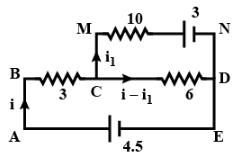Courses

# DC Pandey Test: Current Electricity

## 25 Questions MCQ Test Physics Class 12 | DC Pandey Test: Current Electricity

Description
This mock test of DC Pandey Test: Current Electricity for Class 12 helps you for every Class 12 entrance exam. This contains 25 Multiple Choice Questions for Class 12 DC Pandey Test: Current Electricity (mcq) to study with solutions a complete question bank. The solved questions answers in this DC Pandey Test: Current Electricity quiz give you a good mix of easy questions and tough questions. Class 12 students definitely take this DC Pandey Test: Current Electricity exercise for a better result in the exam. You can find other DC Pandey Test: Current Electricity extra questions, long questions & short questions for Class 12 on EduRev as well by searching above.
QUESTION: 1

### A brass disc and a carbon disc of same radius are assembled alternatively to make a cylindrical conductor. The resistance of the cylinder is independent of the temperature. The ratio of thickness of the brass disc to that of the carbon disc is ______. α is temperature coefficient of resistance & Neglect linear expansion

Solution: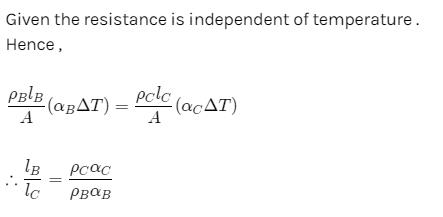QUESTION: 2

### Out of the following which is not a poor conductor ?

Solution: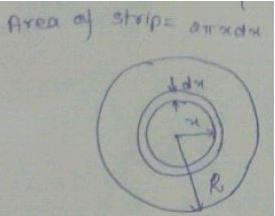J= {J0[(x/R)-1] 0 ≤ x ≤ R/2,     {J0(x/R) R/2 ≤ x ≤ R
We know that,
J=current/area
⇒ current=J Area
Current= J1A1 +J2A2
=J0{(x/R)−1}x(2πxdx)+J0 (x/R)(2πxdx)
=∫0R/2J0{(x/R)-1} x (2πxdx) + =∫0R/2J0 x 2π (x2/R)dx
= J0×2π∫0R/2 {(x2/R)−x}dx + (J0×2π/R) ∫RR/2 x2dx
⇒ i=J0×2π [(x3/3R)-(x2/2)] R/2 + (J0×2π/R) [x3/3]R2/R
⇒i=J0×2π [(R2/24)−(R2/8)]+ (J0×2π/R)[(R3/3)-(R3/24)]
=J0×2π(−2R2/24)(J0×2π/R)[7R3/24]
i =(5/12)πJ0R2

QUESTION: 3

### Under what condition current passing through the resistance R can be increased by short circuiting the battery of emf E2. The internal resistances of the two batteries are r1 and r2 respectively.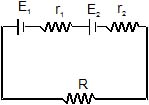Solution:

The current through the circuit before the battery of emf E2​ is short circuited, is
i1​=(E1​+E2)/r1​+r2​+R​​             ...(i)
After short circuiting the battery of emf E2​, current through the resistance R would be
i2​= E1/R+r1​ ​​            ...(ii)
Since, from equation (i) and (ii), we get
i2​>i1​
∴ E1/R+r1​ ​​> E1​+E2​​/R+r1​+r2
This gives E1​r2​>E2​(R+r1​)

QUESTION: 4

ABCD is a square where each side is a uniform wire of resistance 1Ω. A point E lies on CD such that if a uniform wire of resistance 1W is connected across AE and constant potential difference is applied across A and C then B and E are equipotential.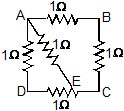Solution:

Wire of resistance =1Ω
Keeping in view that, ABCD is a square where each side is uniform wire of resistance 1Ω. If a uniform wire of resistance 1Ω is connected across AE and a potential difference is applied across A and C, the points B and E will be equipotential; a point E on CD is CE​/ ED =√2/1
∴    CE:ED= √2​:1

QUESTION: 5

Two current elements P and Q have current voltage characteristics as shown below :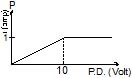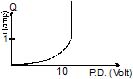Which of the graphs given below represents current voltage characteristics when P and Q are in series.

Solution:
*Multiple options can be correct
QUESTION: 6

A battery is of emf E is being charged from a charger such that positive terminal of the battery is connected to terminal A of charger and negative terminal of the battery is connected to terminal B of charger. The internal resistance of the battery is r.

Solution:

A battery is of emf E is being charged from a charger and hence current inside the cell is from anode to cathode
I=V−E/r​
V=E+Ir
Therefore, when a cell is being charged the potential difference across its terminals is greater than emf of a cell. Also in charging the positive terminal is connected to anode of the cell and negative terminal to cathode.
Here, positive terminal of the battery is connected to terminal A of charger and negative terminal of the battery is connected to terminal B of charger.
Hence, Potential difference across points A and B must be more than E, A must be at higher potential than B and In battery, current flows from positive terminal to the negative terminal

QUESTION: 7

Power of battery in resistor appears as

Solution:
*Multiple options can be correct
QUESTION: 8

Which of the following quantities do not change when a resistor connected to a battery is heated due to the current?

Solution:

Resistance, resistivity and drift velocity varies with relaxation time which is dependent on temperature.
Number of free electrons in a conductor remains invariant even if its temperature changes.
Hence correct option is option D.

*Multiple options can be correct
QUESTION: 9

A metallic conductor of irregular cross-section is as shown in the figure. A constant potential difference is applied across the ends (1) and (2). Then :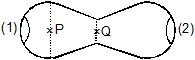Solution:

The answer provided in the forum is wrong,the correct answer would be option A,B,C,D.
Solution:
Current is constant across the cross-section.
E ∝1/A ;     r∝1/A
=>i2r∝1/A
∴heat at Q>heat a P.
∴A,B,C,D are correct.

*Multiple options can be correct
QUESTION: 10

A current passes through a wire of nonuniform cross section. Which of the following quantities are independent of the cross-section?

Solution:

Drift speed and current density are inversely proportional to the area of cross-section of a wire. Thus, they are dependent on the cross-section. The charge crossing in a given time interval is independent of the area of cross-section of the wire. Free electron density is the total number of free electrons per unit volume of the wire. The density of free electrons depends on the distribution of the free electrons throughout the volume of the wire. It does not depend on the cross-section of the wire.

QUESTION: 11

A simple circuit contains an ideal battery and a resistance R. If a second resistor is placed in parallel with the first.

Solution:

When a resistor is added parallel to other resistor in circuit it results in the reduction of overall resistance, since there are multiple pathways by which charge can flow. adding another resistor in a separate branch provides another pathway by which to direct charge through the main area of resistance within the circuit. This decreased resistance resulting from increasing the number of branches will have the effect of increasing the rate at which charge flows i.e. the current.

*Multiple options can be correct
QUESTION: 12

In the circuit shown E, F, G and H are cells of e.m.f. 2V, 1V, 3V and 1V respectively and their internal resistances are 2W, 1W, 3W and 1W respectively.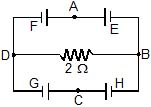Solution: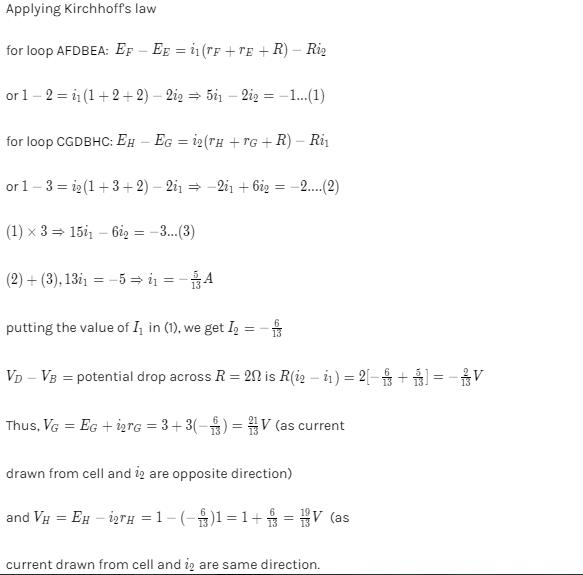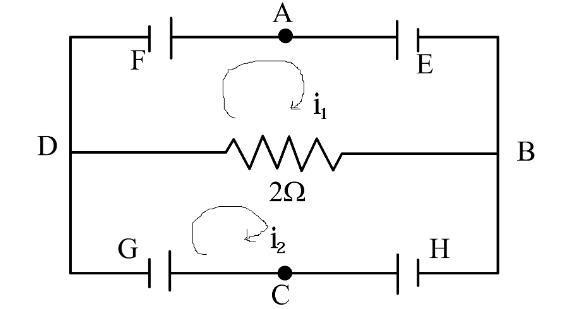QUESTION: 13

Consider the circuit shown in the figure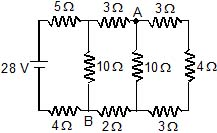Solution: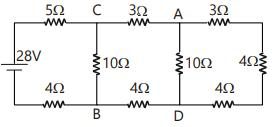RAD=1/(1/10)+(1/3+4+3)=5Ω
RCB=1/(1/10)+(1/3+5+2)= 5Ω
i=28/5+5+4=2A
current through 5Ω is 2A
RCADB=RCB
⇒iCA=iCB=i/2=1A
VCA=1x3=3V
VCB=1x10=10V
VAB=VCB-VCA=10-3
VA-VB=7V

*Multiple options can be correct
QUESTION: 14

The equivalent resistance of a group of resistances is R. If another resistance is connected in parallel to the group, its new equivalent becomes R1 & if it is connected in series to the group, its new equivalent becomes R2 we have

Solution:

The equivalent resistance of the group of resistances is R.
case 1:
a resistance r(say) is connected in parallel to the group, its new equivalent resistance R1 is
R1​=Rr/R+r​
hence, R1​<R.
case 2:
a resistance r is connected in series to the group, its new equivalent resistance R2 is
R2​=R+r
hence, R2​>R.

*Multiple options can be correct
QUESTION: 15

The value of the resistance R in figure is adjusted such that power dissipated in the 2W resistor is maximum. Under this condition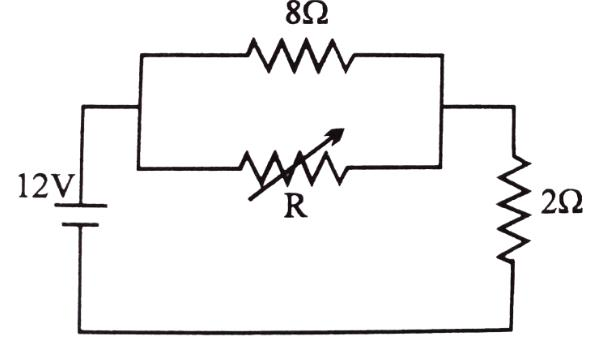Solution:

The power dissipated in circuit is given by
P= V2/R​
For the resistances in parallel the equivalent resistance is less than smallest resistance in combination of resistors hence power dissipation is maximum in this case.
For the resistances in series the equivalent resistance is addition of resistors hence power dissipation is minimum in this case.
Hence, to avoid maximum power dissipation in parallel circuit R must be equal to zero so the equivalent of parallel resistors will be

Rp​=(0)(8)/8+0​=0Ω
Since, Rp​=0Ω voltage across it is zero.
Therefore, power dissipated in 2Ω resistor is
P=V2​/R
P= (12)2​/2
P=72W

*Multiple options can be correct
QUESTION: 16

Statement-1 : When two conducting wires of different resistivity having same cross section area are joined in series, the electric field in them would be equal when they carry current.

Statement-2 : When wires are in series they carry equal current.

Solution:

The electric field does not exist inside the current carrying conductor. Hence, statement 1 is false.
When the wires are in series their cross sectional area is same hence current density will be equal i.e.current through the wires is equal. Thus, statement 2 is correct

*Multiple options can be correct
QUESTION: 17

Statement-1 : Potential difference across the terminals of a battery is always less than its emf.

Statement-2 : A battery always has some internal resistance.

Solution:

The potential difference V across the terminals is not equal to the emf E, but will be smaller because of the voltage drop within the device due to its internal resistance r.If I is current through the circuit then,
V=E−Ir
The resistance offered by medium in between plates of battery (electrolytes and electrodes of the cell) to the flow of current within the battery is called internal resistance of the battery. For an ideal cell r=0 , but real batteries or sources of emf always has same finite internal resistance.

*Multiple options can be correct
QUESTION: 18

Statement-1 : Knowing that rating is done at steady state of the filament, an electric bulb connected to a source having rated voltage consumes more than rated power just after it is switched on.

Statement-2 : When filament is at room temperature its resistance is less than its resistance when the bulb is fully illuminated

Solution:
*Multiple options can be correct
QUESTION: 19

Statement-1 : When a battery is supplying power to a circuit, work done by electrostatic forces on electrolyte ions inside the battery is positive

Statement-2 : Electric field is directed from positive to negative electrode inside a battery

Solution:

When the battery is supplying power, inside the battery positive charge moves opposite to the electric field. So work done by electrostatic forces is negative.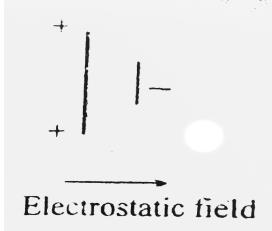Hence, option D is the correct answer.

*Multiple options can be correct
QUESTION: 20

Statement-1 : Conductivity of a metallic conductor decreases with increase in temperature.

Statement-2 : On increasing temperature the number of free electrons in the metallic conductar decreases.

Solution:

On increase in temperature, the number of free electrons increases. But also the collisions will increase. Hence conductivity decreases.

QUESTION: 21

A current of 0.50 ampere is passing through a CuSO4 solution. How many Cu++ions will be deposited on cathode in 10 seconds ?

Solution:

Given that the amount of current passing is 0.5 A. In 10 s time the amount of charge is,
Q=I×t=0.5×10=5 C
Therefore, the number of Cu2+ ions are,
According to quantization,
Q=nq; Where q is the charge of each Cu2+
Therefore,
n=Q/q=5/2e=5/(2×1.6×10−19)=5×1019/3.2
⇒n=1.56×1019

QUESTION: 22

A copper wire of radius 0.1 mm and resistance 1 kW is connected across a power supply of 20 V. How many electrons are transferred per second between the supply and the wire at one end ?

Solution:
QUESTION: 23

A wire has a length of 2.0 m and a resistance of 5.0 W. Find the electric field existing inside the wire if it carries a current of 10 A.

Solution:

A wire has a length =2.0m
resistance =5Ω
Electric field existing inside the wire I=10A
V=I×R
=10×5
=50V
∴   E=50V/2​=25V/m

QUESTION: 24

A car has a fresh storage battery of emf 12 V and internal resistance 5.0 × 10-2W. If the starter draws a current of 90 A, what is the terminal voltage of the battery when the starter is on ?

Solution:
QUESTION: 25

Find the current through the 10 W resistor shown in figure.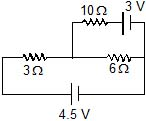Solution:

Applying kvl
first in loop ABCDE
−3i−6(i−i1​) + 4.5=0.                    -------(1)
then in loop ABCMNE
−3i−10i1​−3+4.5=0.                     --------(2)
From equation (1) and (2) WE GET,
i1​=0
hence current passing through 10-ohm resistor is ZERO